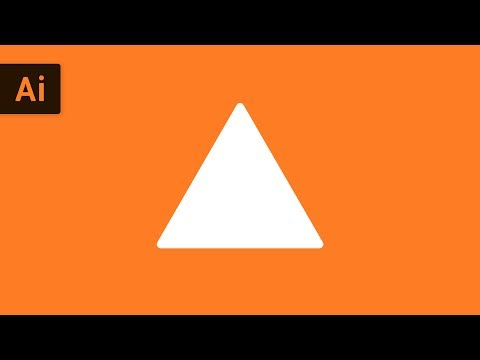# How To Make A Triangle

## Video: How To Make A TriangleVideo: How to Make a Triangle | Illustrator Tutorial 2023, January

If you are into the old Japanese origami technique - the art of folding different shapes out of paper - you need to learn how to fold the basic geometric shapes used in a variety of origami shapes. One of such basic and universal forms, which no origami master can do without, is a double triangle or "water bomb", as it is also called. There are several ways to fold a double triangle.

## Instructions

### Step 1

Take a square piece of paper and fold it along both diagonals. Then flip the square over and fold it in half horizontally, aligning the top and bottom edges.

### Step 2

The folds form the center point of the square. Press on it - the diagonal lines will bend up, and the horizontal line - down. Bend the square along the marked lines. You will have a folded triangle.

### Step 3

Flip its right corner to the left, and then flip the triangle and flip the left corner to the right. This was the first and most common way to fold the basic triangle shape.

### Step 4

Another method to fold the base triangle also uses a square sheet of paper as a base.

### Step 5

Fold the sheet in half by aligning the top with the bottom and bending it towards you. Fold the folded paper in half again along the vertical center line, connecting the right edge to the left.

### Step 6

Fold the square closest to you diagonally towards you, opening the pocket. This will create a front triangle.

### Step 7

Flip the shape over and do the same for the second square, flattening it into a triangle shape.

### Step 8

To fold the base triangle in the third way, fold a square piece of paper diagonally. Turn the resulting triangle with its long base down.

### Step 9

Fold the triangle in half, marking the middle vertical line. Bend the right and left corners of the triangle up, to the center line - one towards you, and one away from you. Flatten the square pockets to create triangles.